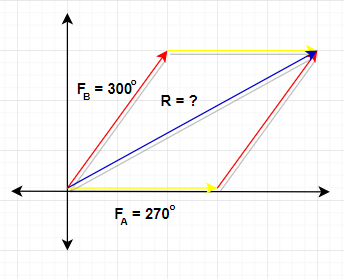# Two dogs pull horizontally on ropes attached to a post; the angle between the ropes is 60.0...

## Question:

Two dogs pull horizontally on ropes attached to a post; the angle between the ropes is 60.0 degrees. If dog A exerts a force of 270N and dog B exerts a force of 300N,

find the magnitude of the resultant force and the angle it makes with dog A's rope.

This problem can be solved using the parallelogram method and this method gives the rule for vector addition of two or more vectors. It is done graphically by placing vector A and B at the same initial point then complete the parallelogram shape.

Given:

• Angle between the ropes : {eq}\theta = 60^{\circ}{/eq}
• Force exerted by Dog A : {eq}F_A = 270\ N{/eq}
• Force exerted by Dog B : {eq}F_B = 300\ N{/eq}

Find:

• Magnitude and direction of the resultant force : {eq}F_R =?{/eq} and {eq}\theta = ?{/eq}

To further understand the question we need to visualize it first using the figure as shown below,(Part 1: Magnitude)

We will use the parallelogram Law of vector addition to solve for the resultant force

\begin{align} F_R^2 &= F_A^2 + 2F_A F_B \cos \theta +F_B^2 \\[0.3cm] F_R &= \sqrt {(270\ \rm N)^2 + 2 (270\ \rm N)(300\ \rm N)(cos(60^{\circ})) + (300\ \rm N)^2) } \\[0.3cm] F_R &= \sqrt{243900 \ \rm N^2} \\[0.3cm] F_R &\approx \boxed{493.9\ \rm N} \end{align}

(Part 2: Direction)

To get the direction, we will use the formula,

\begin{align} \theta &= \tan^{-1}\left(\frac{\sum y}{\sum x} \right) \\[0.3cm] &= \tan^{-1} \left(\frac{F_B \sin \theta}{F_A +F_B \cos \theta} \right) \\[0.3cm] &= \tan^{-1} \left[ \frac{(300\ \rm N) \sin(60^{\circ})}{(270\ \rm N)+(300\ \rm N) \cos(60^{\circ})} \right] \\[0.3cm] &= \tan^{-1} \left(\frac{259.8}{420} \right) \\[0.3cm] &= \tan^{-1}(0.62^{\circ}) \\[0.3cm] &\approx \boxed{31.7^{\circ}} \end{align}